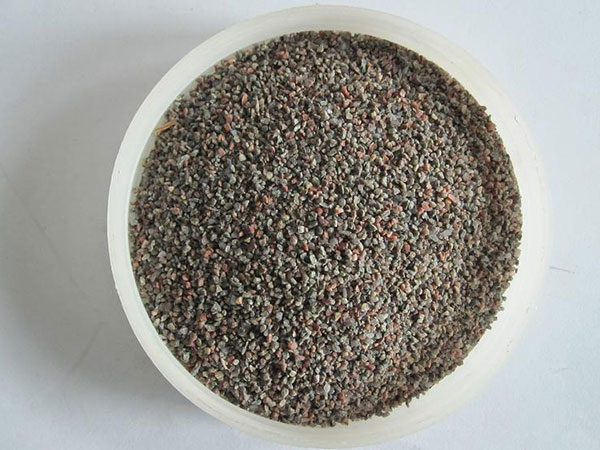# 石家庄嘉瑞矿产品有限公司

15530107555高铝骨料是制作高级耐火材料、耐火砖、浇注料、喷补料、耐火炉料及其它不定型耐火材料的主要原料。

铝矾土熟料按理化指标分为：GAL50，GAL60，GAL70，GAL80，GAL85,GAL88。

铝矾土熟料理化指标

 牌号 化学组成% 体积密度 g/cm3 吸水率 % AL2O3 Fe2O3 Ti02 CaO+MgO K20+Na20 GAL-88 >=88 <=1.5 <=4.0 <=0.4 <=0.4 >=3.15 <=4 GAL-85 >=85 <=1.8 <=4.0 <=0.4 <=0.4 >=3.10 <=4 GAL-80 >=80 <=2.0 <=4.0 <=0.5 <=0.5 >=2.90 <=5 GAL-70 >70--80 <=2.0 - <=0.6 <=0.6 >=2.75 <=5 GAL-60 >60--70 <=2.0 - <=0.6 <=0.6 >=2.65 <=6 GAL-50 >50--60 <=2.5 - <=0.6 <=0.6 >=2.45 <=6